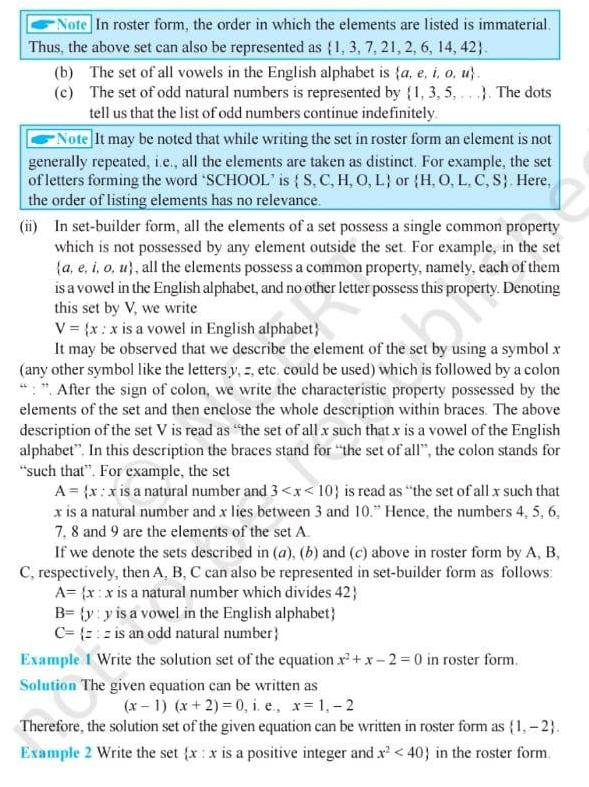Question:

# Note In roster form the order in which the elements are

Last updated: 9/19/2023Note In roster form the order in which the elements are listed is immaterial Thus the above set can also be represented as 1 3 7 21 2 6 14 42 b The set of all vowels in the English alphabet is a e i o u c The set of odd natural numbers is represented by 1 3 5 The dots tell us that the list of odd numbers continue indefinitely Note It may be noted that while writing the set in roster form an element is not generally repeated i e all the elements are taken as distinct For example the set of letters forming the word SCHOOL is S C H O L or H O L C S Here the order of listing elements has no relevance ii In set builder form all the elements of a set possess a single common property which is not possessed by any element outside the set For example in the set a e i o u all the elements possess a common property namely each of them is a vowel in the English alphabet and no other letter possess this property Denoting this set by V we write P V x x is a vowel in English alphabet It may be observed that we describe the element of the set by using a symbol x any other symbol like the letters y z etc could be used which is followed by a colon After the sign of colon we write the characteristic property possessed by the elements of the set and then enclose the whole description within braces The above description of the set V is read as the set of all x such that x is a vowel of the English alphabet In this description the braces stand for the set of all the colon stands for such that For example the set A x x is a natural number and 3 x 10 is read as the set of all x such that x is a natural number and x lies between 3 and 10 Hence the numbers 4 5 6 7 8 and 9 are the elements of the set A If we denote the sets described in a b and c above in roster form by A B C respectively then A B C can also be represented in set builder form as follows A x x is a natural number which divides 42 B y y is a vowel in the English alphabet C is an odd natural number Example 1 Write the solution set of the equation x x 2 0 in roster form Solution The given equation can be written as x 1 x 2 0 i e x 1 2 Therefore the solution set of the given equation can be written in roster form as 1 2 Example 2 Write the set x x is a positive integer and x 40 in the roster form